# Dispersion-Managed Solitons

Dispersion management is employed commonly for modern WDM systems. Since such systems use fiber sections with both normal and anomalous GVDs, one may ask what happens to solitons in this case. It turns out that solitons can form even when the GVD parameter β2 varies along the link length but their properties are quite different. This tutorial is devoted to such dispersion-managed solitons. We first consider dispersion-decreasing fibers and then focus on fiber links with periodic dispersion maps.

#### 1. Dispersion-Decreasing Fibers

An interesting scheme relaxes completely the restriction LA ≪ LD imposed normally on loss-managed solitons by employing a new kind of fiber in which GVD varies along the fiber length. Such fibers are called dispersion-decreasing fibers (DDFs) and are designed such that the decreasing GVD counteracts the reduced SPM experienced by solitons weakened from fiber losses.

Soliton evolution in a DDF is governed by the NLS equation except that β2 is a continuous function of z. Introducing the normalized distance and time variables as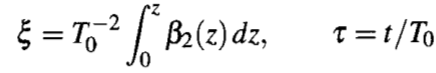we can write this equation in the form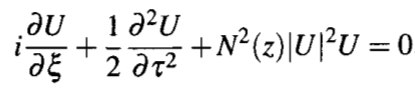where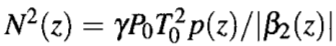If the GVD profile is chosen such that |β2(z)| = |β2(0)|p(z), N becomes a constant, and the NLS equation above reduces the standard NLS equation obtained earlier with p(z) = 1. As a result, fiber losses have no effect on a soliton in spite of its reduced energy when DDFs are used. More precisely, lumped amplifiers can be placed at any distance and are not limited by the condition LA ≪ LD, provided the GVD decreases exponentially in the fiber section between two amplifiers asThis result can be understood qualitatively by noting that the requirement N = 1 can be maintained, in spite of power losses, if both |β2| and γ decrease exponentially at the same rate. The fundamental soliton then maintains its shape and width even in a lossy fiber.

Fibers with a nearly exponential GVD profile have been fabricated. A practical technique for making such DDFs consists of reducing the core diameter along the fiber length in a controlled manner during the fiber-drawing process. Variations in the fiber diameter change the waveguide contribution to β2 and reduce its magnitude. Typically, GVD can be varied by a factor of 10 over a length of 20 to 40 km. The accuracy realized by the use of this technique is estimated to be better than 0.1 ps2/km. The propagation of solitons in DDFs has been observed in several experiments. In a 40-km DDF, solitons preserved their width and shape in spite of energy losses of more than 8 dB. In a recirculating loop made using DDFs, a 6.5-ps soliton train at 10 Gb/s could be transmitted over 300 km.

In an alternative approach, the exponential GVD profile of a DDF is approximated with a staircase profile by splicing together several constant-dispersion fibers with different β2 values. This approach was studied during the 1990s, and it was found that most of the benefits of DDFs can be realized using as few as four fiber segments. How should one select the length and the GVD of each fiber used for emulating a DDF? The answer is not obvious, and several methods have been proposed. In one approach, power deviations are minimized in each section. In another approach, fibers of different GVD values Dm and different lengths Lmap are chosen such that the product  DmLmap is the same for each section. In a third approach, Dm and Lmap are selected to minimize the shading of dispersive waves. Advantages offered by DDFs for soliton systems include a lower timing jitter and a reduced noise level. In spite of these benefits, DDFs are rarely used in practice.

#### 2. Periodic Dispersion Maps

Periodic dispersion maps consisting of alternating-GVD fibers are attractive because their use lowers the average dispersion of the entire link, while keeping the GVD of each section large enough that the FWM crosstalk remains negligible in WDM systems. However, the use of dispersion management forces each soliton to propagate in the normal-dispersion regime of a fiber during each map period. At first sight, such a scheme should not even work because the normal-GVD fibers do not support solitons and lead to considerable broadening and chirping of the pulse. So, why should solitons survive in a dispersion-managed fiber link? An intense theoretical effort devoted to this issue led to the discovery of dispersion-managed (DM) solitons. Physically speak, if the dispersion length associated with each fiber section used to form the map is a fraction of the nonlinear length, the pulse would evolve in a linear fashion over a single map period. On a longer length scale, solitons can still form if the SPM effects are balanced by the average dispersion. As a result, solitons can survive in an average sense, even though not only the peak power but also the width and shape of such solitons oscillate periodically.

We focus on a simple dispersion map consisting of two fibers with opposite GVD characteristics. Soliton evolution is governed by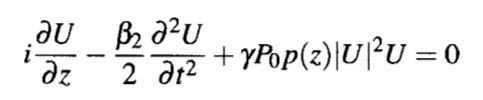in which β2 is a piecewise continuous function of z taking values β2a and β2n in the anomalous and normal GVD sections of lengths la and ln, respectively. The map period Lmap = la + ln can be different from the amplifier spacing LA. Clearly, the properties of DM solitons will depend on several map parameters even when only two types of fibers are used in each map period. Numerical simulations show that a nearly periodic solution can often be found by adjusting input pulse parameters (width, chirp, and peak power) even though these parameters vary considerably in each map period. The shape of such DM solitons is closer to a Gaussian profile rather than the "sech" shape associated with standard solitons.

Numerical solutions, although essential, do not lead to much physical insight. Several techniques have been used to solve the NLS equation approximately. A common approach makes use of the variational method. Another approach expands B(z, t) in terms of a complete set of the Hermite-Gauss functions that are solutions of the linear problem. A third approach solves an integral equation, derived in the spectral domain using perturbation theory.

Here we focus on the variational equations. Because the shape of DM solitons is close to a Gaussian pulse, they can be applied for them. These two equations should be solved with the periodic boundary conditions to ensure that the DM soliton recovers its initial state after each amplifier. The periodic boundary conditions fix the values of the initial width T0 and the chirp C0 at z = 0 for which a soliton can propagate in a periodic fashion for a given value of pulse energy E0. A new feature of the DM solitons is that the input pulse width depends on the dispersion map and cannot be chosen arbitrarily. In fact, T0 cannot fall below a critical value that is set by the map itself.

The following figure shows how the pulse width T0 and the chirp C0 of allowed periodic solutions vary with input pulse energy for a specific dispersion map.The minimum value Tm of the pulse width occurring in the middle of the anomalous-GVD section of the map is also shown. The map is suitable for 40-Gb/s systems and consists of alternating fibers with GVD of -4 and 4 ps2/km and lengths la ≈ ln = 5 km such that the average GVD is -0.01 ps2/km. The solid lines show the case of ideal distributed amplification for which p(z) = 1. The lumped amplification case is shown in the dashed lines, assuming 80-km amplifier spacing and 0.25 dB/km losses in each fiber section.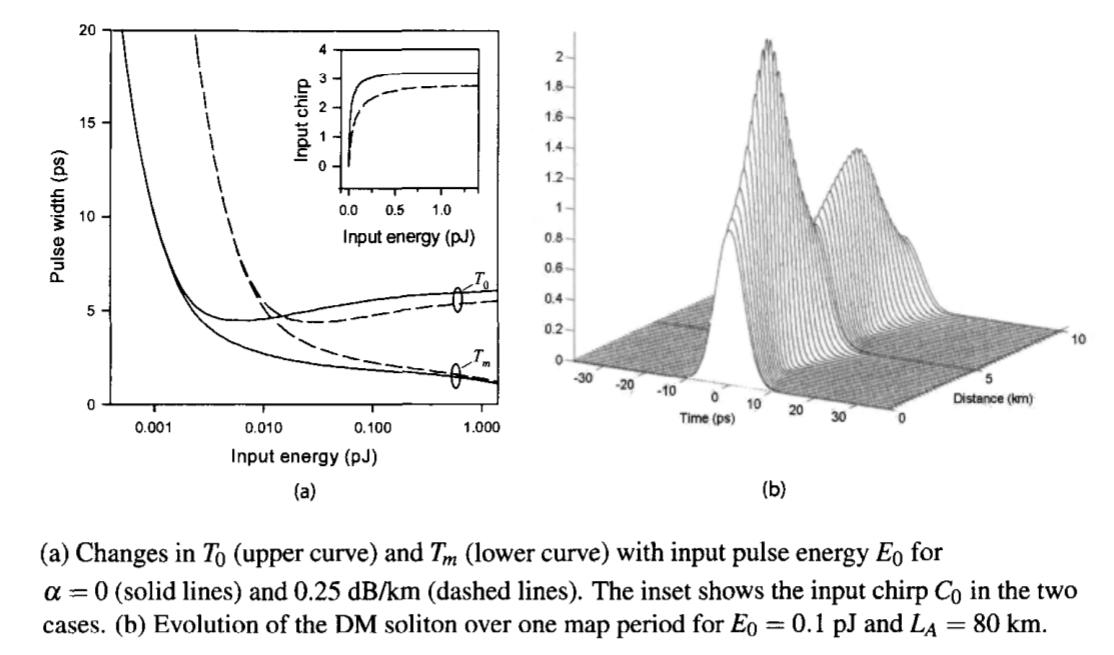Several conclusions can be drawn from the figure above. First, both T0 and Tm decrease rapidly as pulse energy is increased. Second, T0 attains its minimum value at a certain pulse energy Ec while Tm keeps decreasing slowly. Third, T0 and Tm differ by a large factor for E0 ≫ Ec. This behavior indicates that pulse width changes considerably in each fiber section when this regime is approached. An example of pulse breathing is shown in figure (b) above for E0 = 0.1 pJ in the case of lumped amplification. The input chirp C0 is relatively large (C0 ≈ 1.8) in this case. The most important feature of the figure above is the existence of a minimum value of T0 for a specific value of the pulse energy. The input chirp C0 = 1 at that point. It is interesting to note that the minimum value of T0 does not depend much on fiber losses and is about the same for the solid and dashed curves, although the value of Ec is much larger in the lumped amplification case because of fiber losses.

Both the pulse width and the peak power of DM solitons vary considerably within each map period. Figure (a) below shows the width and chirp variations over one map period for the DM soliton of figure (b) above. The pulse width varies by more than a factor of 2 and becomes minimum nearly in the middle of each fiber section where frequency chirp vanishes. The shortest pulse occurs in the middle of the anomalous-GVD section in the case of ideal distributed amplification in which fiber losses are compensated fully at every point along the fiber link. For comparison, figure (b) below shows the width and chirp variations for a DM soliton whose input energy is close to Ec where the input pulse is shortest. Breathing of the pulse is reduced considerably together with the range of chirp variations. In both cases, the DM soliton is quite different from a standard fundamental soliton as it does not maintain its shape, width, or peak power. Nevertheless, its parameters repeat from period to period at any location within the map. For this reason, DM solitons can be used for optical communications in spite of oscillations in the pulse width. Moreover, such solitons perform better from a system standpoint.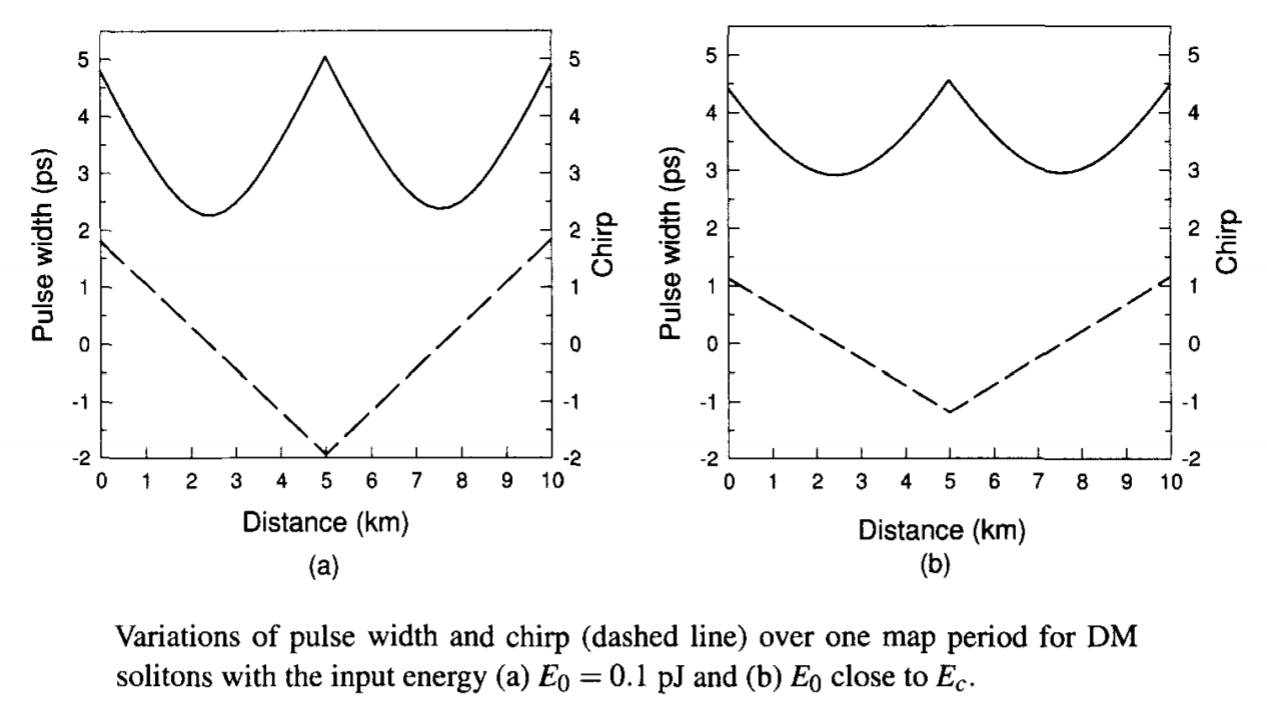#### 3. Design Issues

The two figures above show that the equations permit periodic propagation of many different DM solitons in the same map by choosing different values of E0, T0, and C0. How should one choose among these solutions when designing a soliton system? Pulse energies much smaller than Ec (corresponding to the minimum value of T0) should be avoided because a low average power would then lead to rapid degradation of SNR as amplifier noise builds up with propagation. On the other hand, when E0 ≫ Ec, large variations in the pulse width in each fiber section would induce XPM-induced interaction between two neighboring solitons if their tails begin to overlap considerably. For this reason, the region near E0 = Ec is most suited for designing DM soliton systems. Numerical solutions of the NLS equation confirm this conclusion.

The 40-Gb/s system design used for the two figures above was possible only because the map period Lmap was chosen to be much smaller than the amplifier spacing of 80 km, a configuration referred to as the dense dispersion management. When Lmap is increased to 80 km using la ≈ ln = 40 km, while keeping the same value of average dispersion, the minimum pulse width supported by the map increases by a factor 3. The bit rate is then limited to below 20 Gb/s.

It is possible to find the values of T0 and Tm by solving the NLS equations approximately. The equation shows that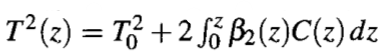at any point within the map. The chirp equation cannot be integrated analytically but the numerical solutions show that C(z) varies almost linearly in each fiber section. As seen in the figure above, C(z) changes from C0 to -C0 in the first section and then back to C0 in the second section. Noting that the ratio (1 + C2)/T2 is related to the spectral width that changes little over one map period when the nonlinear length is much larger than the local dispersion length, we average it over one map period and obtain the following relation between T0 and C0: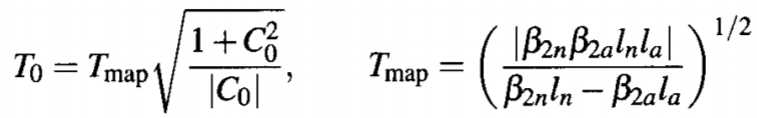where Tmap is a parameter with dimensions of time involving only the four map parameters. It provides a time scale associated with an arbitrary dispersion map in the sense that the stable periodic solutions supported by it have input pulse widths that are close to Tmap (within a factor of 2 or so). The minimum value of T0 occurs for |C0| = 1 and is given by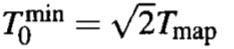It is useful to look for other combinations of the four map parameters that may play an important role in designing a DM soliton system. Two parameters that are useful for this purpose are defined as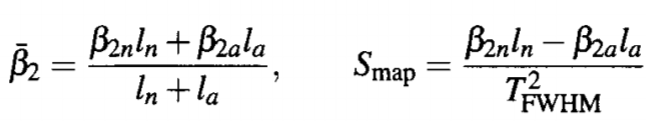where TFWHM ≈ 1.665Tm is the FWHM at the location where pulse width is minimum in the anomalous-GVD section. Physically,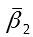represents the average GVD of the entire link, while the map strength Smap is a measure how much GVD changes abruptly between two fibers in each map period. The solutions as a function of map strength S for different values ofreveal the surprising feature that DM solitons can exist even when the average GVD is normal, provided the map strength exceeds a critical value Scr.

As an example, the following figure shows DM-soliton solutions for several values of Smap by plotting peak power as a function of the dimensionless ratio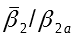. Periodic solutions in the normal-GVD regime exist only when Smap exceeds a critical value of 4.8, indicating that pulse width for such solutions changes by a large factor in each fiber section. Moreover, when Smap > Scr, a periodic solution can exist for two different values of the input pulse energy in a small range of positive values of> 0. Numerical solutions confirm these predictions, except that the critical value of the map strength is found to be 3.9.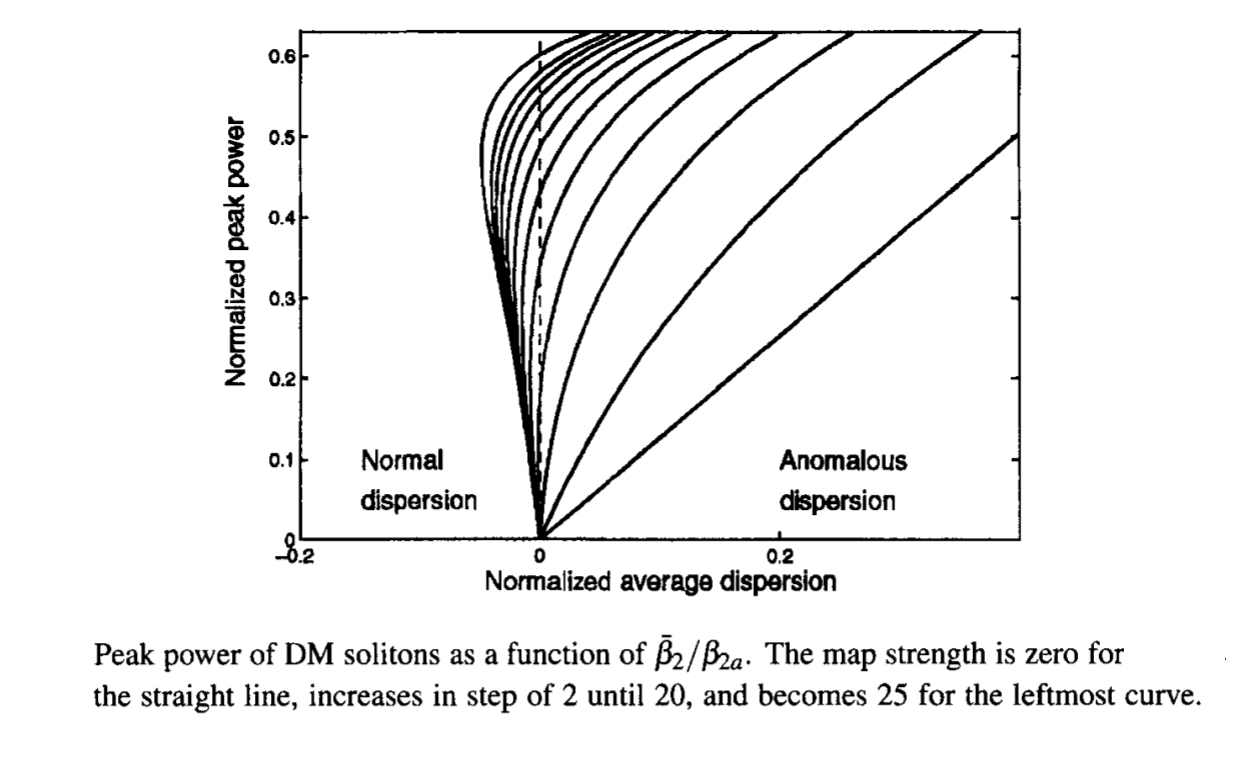For map strengths below a critical value (about 3.9 numerically), the average GVD is anomalous for DM solitons. In that case, one is tempted to compare them with standard solitons forming in a uniform-GVD fiber link with. For relatively small values of Smap, variations in the pulse width and chirp are small enough that one can ignore them. The main difference between the average-GVD and DM solitons then stems from the higher peak power required to sustain DM solitons. The energy enhancement factor for DM solitons is defined as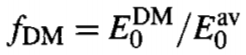and can exceed 10 depending on the system design. The larger energy of DM solitons benefits a soliton system in several ways. Among other things, it improves the SNR and decreases the timing jitter.

Dispersion-management schemes were used for solitons as early as 1992 although they were referred to by names as partial soliton communication and dispersion allocation. In the simplest form of dispersion management, a relatively short section segment of dispersion-compensating fiber (DCF) is added periodically to the transmission fiber, resulting in dispersion maps similar to those used for nonsoliton systems. It was found in a 1995 experiment that the use of DCFs reduced the timing jitter considerably. In fact, in this 20-Gb/s experiment, the timing jitter became low enough when the average dispersion was reduced to a value near -0.025 ps2/km that the 20-Gb/s signal could be transmitted over transoceanic distances.

A large number of experiments have shown the benefits of DM solitons for lightwave systems. In one experiment, the use of a periodic dispersion map enabled the transmission of a 20-Gb/s soliton bit stream over 5,520 km of a fiber link containing amplifiers at 40-km intervals. In another 20-Gb/s experiment, solitons could be transmitted over 9,000 km without using any in-line optical filters since the periodic use of DCFs reduced timing jitter by more than a factor of 3. A 1997 experiment focuses on the transmission of DM solitons using dispersion maps such that solitons propagated most of the time in the normal-GVD regime. This 10-Gb/s experiment transmitted signals over 28,000 km using a recirculating fiber loop consisting of 100 km of normal-GVD fiber and 8 km of anomalous-GVD fiber such that the average GVD was anomalous (about -0.1 ps2/km). Periodic variations in the pulse width were also observed in such a fiber loop. In a later experiment, the loop was modified to yield the average-GVD value of zero or a slightly positive value. Stable transmission of 10-Gb/s solitons over 28,000 km was still observed. In all cases, experimental results were in excellent agreement with those of numerical simulations.

An important application of dispersion management consists of upgrading the existing terrestrial networks designed with standard fibers. A 1997 experiment used fiber gratings for dispersion compensation and realized 10-Gb/s soliton transmission over 1,000 km. Longer transmission distances were realized using a recirculating fiber loop consisting of 102 km of standard fiber with anomalous GVD (β2 ≈ -21 ps2/km) and 17.3 km of DCF with normal GVD (β2 ≈ 160 ps2/km). The map strength S was quite large in this experiment when 30-ps (FWHM) pulses were launched into the loop. By 1999, 10-Gb/s DM solitons could be transmitted over 16,000 km of standard fiber when soliton interactions were minimized by choosing the location of amplifiers appropriately.

#### 4. Timing Jitter

We saw in this role of dispersive and nonlinear effects tutorial that noise added by optical amplifiers perturbs the position of each optical pulse in its time slot. Timing jitter induced by amplifier noise is especially severe for soliton-based systems and it limits the total transmission distance of any long-haul soliton link. This limitation was first pointed out in 1986 in the context of standard constant-width solitons. It persists even for DM soliton systems, although jitter is reduced for them. In all cases, the dominant source of timing jitter is related to changes in the soliton carrier frequency, occurring because of phase fluctuations induced by amplifier noise.

The moment method can be used to calculate the variance of fluctuations in the frequency and pulse position. Since pulse shape remains approximately Gaussian for DM solitons, the analysis applies for them with minor changes. The final result can be written as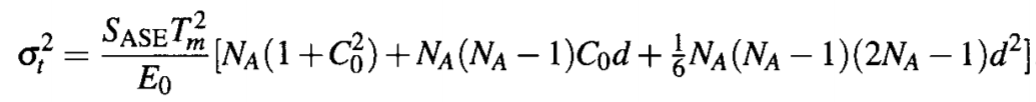where NA is the number of amplifiers along the link and the dimensionless parameter d is defined, except that the minimum width Tm should be used in place of T0. The first term inside the square brackets results from direct position fluctuations of a soliton within each amplifier. The second term is related to the cross-correlation between frequency and position fluctuations. The third term is solely due to frequency fluctuations. For a soliton system designed with Lmap = LA and NA ≫ 1, jitter is dominated by the last term because of its NA3 dependence and is approximately given bywhere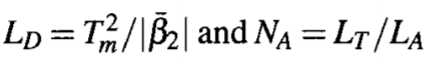for a lightwave system with the total transmission distance LT.

Because of the cubic dependence of σt2 on the system length LT, the timing jitter can become an appreciable fraction of the bit slot for long-haul systems, especially at bit rates exceeding 10 Gb/s for which the bit slot is shorter than 100 ps. Such jitter would lead to large power penalties if left uncontrolled. In practice, jitter should be less than 10% of the bit slot. The figure below shows how timing jitter increases with LT for a 20-Gb/s DM soliton system, designed using a dispersion map consisting of 10.5 km of anomalous-GVD fiber and 9.7 km of normal-GVD fiber [D = ±4 ps/(km-nm)]. Optical amplifiers with nsp = 1.3 (or a noise figure of 4.1 dB) are placed every 80.8 km (4 map periods) along the fiber link for compensating 0.2-dB/km losses. Variational equations were used to find the input pulse parameters for which solitons recover periodically after each map period (T0 = 6.87 ps, C0 = 0.56, and E0 = 0.4 pJ). The nonlinear parameter γ was 1.7 W-1/km.An important question is whether the use of dispersion management is helpful or harmful from the standpoint of timing jitter. The timing jitter for standard solitons can also be found in a closed form using the moment method and is given bywhere we have used Es for the input soliton energy to emphasize that it is different from the DM soliton energy E0. For a fair comparison of the DM and standard solitons, we consider an identical soliton system except that the dispersion map is replaced by a single fiber whose GVD is constant and equal to the average value. The soliton energy Es can be found by using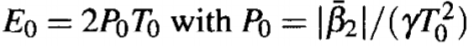and is given bywhere the factor fLM is the enhancement factor resulting from loss management (fLM ≈ 3.8 for a 16-dB gain). The dashed line in the figure above shows the timing jitter using the two equations above. A comparison of the two curves shows that the jitter is considerably smaller for DM solitons. The physical reason behind the jitter reduction is related to the enhanced energy of the DM solitons. In fact, the energy ratio E0/Es equals the energy enhancement factor fDM introduced earlier. From a practical standpoint, the reduced jitter of DM solitons permits much longer transmission distance as evident from the figure above. Note that the equation above also applies for DDFs because the GVD variations along the fiber can be included through the parameter d.

For long-haul solitons systems, the number of amplifiers is large enough that the NA3 term dominates, and the timing jitter for standard soliton is approximately given byComparing the two equations, we find that timing jitter is reduced by a factor of (fDM/3)1/2 when DM solitons are used.

To find a simple design rule, we use this equation with the condition σt < bj/B, where bj is the fraction of the bit slot by which a soliton can move without affecting the system performance adversely. Using B = (2q0T0)-1 and Es, the bit rate-distance product BLT for standard solitons is found to be limited byFor DM solitons the energy enhancement factor fLM is replaced by fLMfDM/3. The tolerable value of bj depends on the acceptable BER and on details of the receiver design; typically, bj < 0.1. To see how amplifier noise limits the total transmission distance, consider a standard soliton system operating at 10 Gb/s with parameter values T0 = 10 ps, q0 = 5, α = 0.2 dB/km, γ = 2 W-1/km,= -1 ps/(km-nm), nsp = 1.5, LA = 50 km, and bj = 0.08. Using G = 10 dB, we find fLM = 2.56 and SASE = 2.16 x 10-6 pJ. With these values, BLT must be below 70 (Tb/s)-km, and the transmission distance is limited to 7,000 km at 10 Gb/s. This value can be increased to beyond 10,000 km for DM solitons.

#### 5. Control of Timing Jitter

As the timing jitter ultimately limits the performance of a soliton system, it is essential to find a solution to the timing-jitter problem before the use of solitons can become practical. Several techniques were developed during the 1990s for controlling timing jitter. This subsection is devoted to a brief discussion of them.

The use of optical filters for controlling the timing jitter of solitons was proposed as early as 1991. This approach makes use of the fact that the ASE occurs over the entire amplifier bandwidth but the soliton spectrum occupies only a small fraction of it. The bandwidth of optical filters is chosen such that the soliton bit stream passes through the filter but most of the ASE is blocked. If an optical filter is placed after each amplifier, it improves the SNR because of the reduced ASE and also reduces the timing jitter simultaneously. This was indeed found to be the case in a 1991 experiment but the reduction in timing jitter was less than 50%.

The filter technique can be improved dramatically by allowing the center frequency of the successive optical filters to slide slowly along the link. Such sliding-frequency filters avoid the accumulations of ASE within the filter bandwidth and, at the same time, reduce the growth of timing jitter. The physical mechanism behind the operation of such filters can be understood as follows. As the filter passband shifts, solitons shift their spectrum as well to minimize filter-induced losses. In contrast, the spectrum of ASE cannot change. The net result is that the ASE noise accumulated over a few amplifiers is filtered out later when the soliton spectrum has shifted by more than its own bandwidth.

The moment method can be extended to include the effects of optical filters by noting that each filter modifies the soliton field such that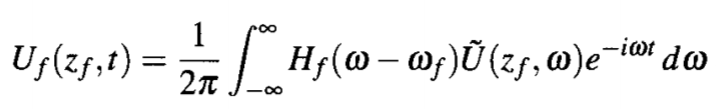whereis the pulse spectrum and Hf is the transfer function of the optical filter located at zf. The filter bandwidth is shifted by ωf from the soliton carrier frequency. If we approximate the filter spectrum by a parabola over the soliton spectrum and use Hf(ω - ωf) = 1 - b(ω - ωf)2, it is easy to see that the filter introduces an additional loss for the soliton that should be compensated by increasing the gain of optical amplifiers. The analysis of timing jitter shows that sliding-frequency filters reduce jitter considerably for both the standard and DM solitons.

Solitons can also be controlled in the time domain using the technique of synchronous amplitude modulation, implemented in practice using a LiNbO3 modulator. The technique works by introducing additional losses for those solitons that have shifted from their original potion (center of the bit slot). The modulator forces solitons to move toward its transmission peak where the loss is minimum. Mathematically, the action of the modulator is to change the soliton amplitude as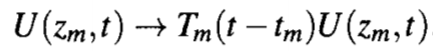where Tm(τ) is the transmission coefficient of the modulator located at z = zm. The moment method or perturbation theory can be used to show that timing jitter is reduced considerably by modulators.

The synchronous modulation technique can also be implemented by using a phase modulator. One can understand the effect of periodic phase modulation by recalling that a frequency shift is associated with all time-dependent phase variations. Since a change in soliton frequency is equivalent to a change in the group velocity, phase modulation induces a temporal displacement. Synchronous phase modulation is implemented in such a way that the soliton experiences a frequency shift only if it moves away from the center of the bit slot, which confines it to its original position despite the timing jitter induced by ASE and other sources. Intensity and phase modulations can be combined together to further improve system performance.

Synchronous modulation can be combined with optical filters to control solitons simultaneously in both the time and frequency domains. In fact, this combination permits arbitrarily long transmission distances. The use of intensity modulators also permits a relatively large amplifier spacing by reducing the impact of dispersive waves. This property of modulators was exploited in 1995 to transmit a 20-Gb/s soliton train over 150,000 km with an amplifier spacing of 105 km. Synchronous modulators also help in reducing the soliton interaction and in clamping the level of amplifier noise. The main drawback of modulators is that they require a clock signal that is synchronized with the original bit stream.

A relatively simple approach uses post-compensation of accumulated dispersion for reducing timing jitter. The basic idea can be understood from the equations obtained for the timing jitter of DM and standard solitons. The cubic term that dominates the jitter at long distances depends on the accumulated dispersion through the parameter d. If a fiber is added at the end of the link such that it reduces the accumulated dispersion, it should help in reducing the jitter. It is easy to include the contribution of the post-compensation fiber to the timing jitter using the moment method. In the case of DM solitons, the jitter variance at the end of a post-compensation fiber of length Lc and GVD β2c is given by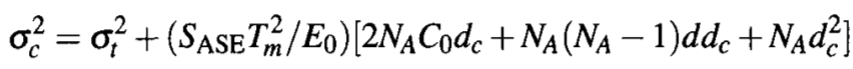whereIf we define y = - dc/(NAd) as the fraction by which the accumulated dispersion NAd is compensated and retain only the dominant cubic terms, this equation can be written asThe minimum value occurs for y = 0.5 for which σc2 is reduced by a factor 4. Thus, the timing jitter of solitons can be reduced by a factor of 2 by postcompensating the accumulated dispersion by 50%. The same conclusion holds for standard solitons.

Several other techniques can be used for controlling timing jitter. One approach consists of inserting a fast saturable absorber periodically along the fiber link. Such a device absorbs low-intensity light, such as ASE and dispersive waves, but leaves the solitons intact by becoming transparent at high intensities. To be effective, it should respond at a time scale shorter than the soliton width. It is difficult to find an absorber that can respond at such short time scales. A nonlinear optical loop mirror can act as a fast saturable absorber and reduce the timing jitter of solitons, while also stabilizing their amplitude. Re-timing of a soliton train can also be accomplished by taking advantage of cross-phase modulation. The technique overlaps the soliton data stream and another pulse train composed of only 1 bits (an optical clock) inside a fiber where cross-phase modulation (XPM) induces a nonlinear phase shift on each soliton in the signal bit stream. Such a phase modulation translates into a net frequency shift only when the soliton does not lie in the middle of the bit slot. Similar to the case of synchronous phase modulation, the direction of the frequency shift is such that the soliton is confined to the center of the bit slot. Other nonlinear effects such as stimulated Raman scattering and four-wave mixing (FWM) can also be exploited for controlling the soliton parameters. The technique of distributed amplification also helps in reducing timing jitter.